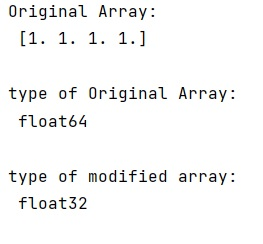# How to convert NumPy array type and values from Float64 to Float32?

Given a NumPy array whose type and values are in Float64, we have to convert them into Float32.
Submitted by Pranit Sharma, on February 22, 2023

NumPy is an abbreviated form of Numerical Python. It is used for different types of scientific operations in python. Numpy is a vast library in python which is used for almost every kind of scientific or mathematical operation. It is itself an array which is a collection of various methods and functions for processing the arrays.

## Converting NumPy array type and values from Float64 to Float32

Suppose that we are given a numpy array of type Float64 and we need to convert this array into Float32 type.

For this purpose, we need to perform type conversion of the numpy array. We will calculate a Float32 variable and put it as an entry into a Float64 numpy array. numpy then converts it properly back to Float64.

Let us understand with the help of an example,

## Python code to convert NumPy array type and values from Float64 to Float32

```# Import numpy
import numpy as np

# Creating a numpy array
arr = np.ones(4,dtype="float64")

# Display original array
print("Original Array:\n",arr,"\n")

# Display type of original array
print("type of Original Array:\n",arr.dtype,"\n")

# Converting array into float32
arr = np.float32(arr)

# Display type of modified array
print("type of modified array:\n",arr.dtype,"\n")
```

Output: